# Survival analysis in R (Kaplan–Meier, Cox proportional hazards, and Log-rank test methods)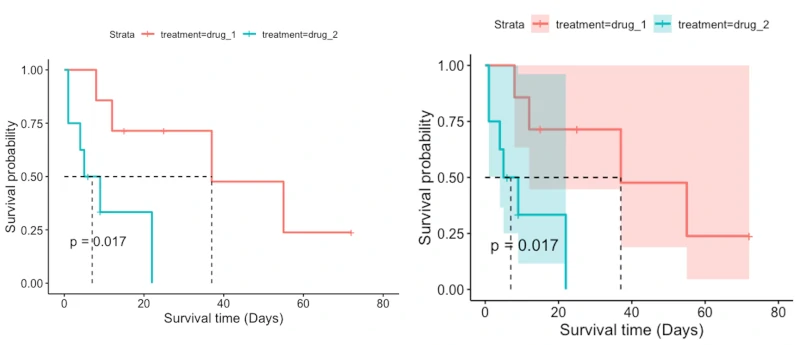## Introduction to survival analysis

• Survival analysis (time-to-event analysis) is a collection of statistical methods for analyzing the duration of time (survival time) until the occurrence of the event of interest (e.g. death or relapse).
• Survival time is a follow-up time measured between the defined starting point and occurrence of an event of interest e.g. time from disease diagnosis and death.
• Some patients’ survival times may not be known in survival analysis. This is because not all patients are exposed to the event of interest, and some patients are lost to follow-up before the study period ends. This phenomenon is known as censoring.
• The standard statistical methods can not be applied to analyze survival data as the data is often censored, heavily skewed, and not normally distributed. These features of survival data makes survival analysis unique method and special statistical methods used for survival analysis.
• The main goal of survival analysis is to estimate the survival probability from survival time and assess the effect of any confounding factors on survival time.
• Survival analysis mostly used in cancer studies. In clinical research, the survival analysis is widely used for predicting the time to death.

## Common terms in Survival analysis

Two time-dependent functions viz. survival function S(t) and the hazard function h(t) are important terms in survival analysis.

Survival function (survival probability), S(t), refers to a probability of surviving of a patient from the starting time ( e.g. start of diagnosis) to beyond a specific time t. Survival function also known as survivor function.

Hazard function or hazard rate, h(t), refers to the instantaneous rate of occurrence of event of interest given that the patient is survived until that time. The higher value of hazard function represents higher risk of event of interest. The hazard function is a crucial part of Cox proportional hazards (CPH) model (also known as Cox regression).

Survival function represents the probability of not having an event of interest, whereas hazard function represents the probability of an event of interest occurring.

Hazard ratio (HR) is similar to relative risk and is described as the ratio of hazard rate or failure rate in two treatment groups (e.g. treated vs. control group). The HR = 1 indicates that there are no differences between the two groups. HR > 1 indicates that the event of interest is most likely to occur and vice versa.

## Methods of survival analysis

### Kaplan–Meier method

Kaplan–Meier method is a nonparametric method for survival analysis. It assumes no specific distribution of survival times and does not assume a relationship between survival times and independent variables.

Kaplan–Meier method estimates the survival probability from the observed survival times (both censored and uncensored). Survival probability is plotted against time t in Kaplan–Meier survival curve. The survival curve is useful for understanding the median survival time (time at which survival probability is 50%).

Kaplan–Meier method is suitable for simple survival analysis and does not consider other independent variables (confounding factors) while analyzing survival curves. If there are other confounding factors that you want to include in model, you should use Cox proportional hazards (PH) model (Cox regression).

Kaplan–Meier survival function is given as,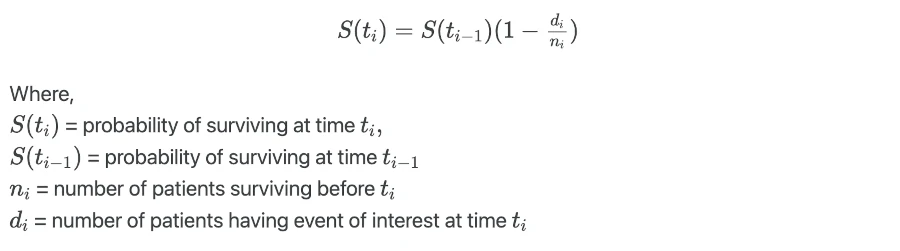The estimated survival probability only changes at the time (ti) of the event of interest, and it is constant between the two events (ti and ti+1). At time zero (where study begins), the survival probability is 1 (S(0)=1) i.e. all patients are alive at time zero.

The flat survival curve (which is inclined to 1) is good, but vertical survival curve which drops toward 0 is bad (suggest poor survival).

If there are a large number of censored patients in the study, the survival curve may not be reliable. The results should be interpreted cautiously.

### Log-rank test

Log-rank test is a nonparametric hypothesis test, which compares two or more survival curves (i.e. survival times for two different condition groups).

The hypothesis for Log-rank test is given as,
Null hypothesis: there are no differences in the survival curves between group1 and group2
Alternate hypothesis: there are differences in the survival curves between group1 and group2

Log-rank test calculates the test statistics by comparing the observed number of events to the expected number of events in underlying condition groups,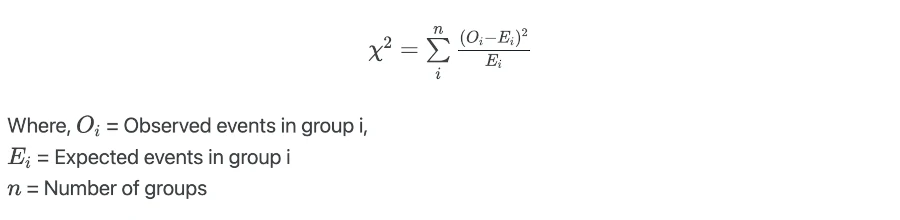Log-rank test value is compared against the critical value from χ2 distribution with n-1 degree of freedoms

The drawback of Log-rank test is that it does not analyze other independent variables affecting the survival time.

### Cox proportional hazards (CPH) model (Cox regression)

Cox proportional hazards (CPH) model is a semiparametric model. It analyzes multiple independent variables for estimating differences between the survival curves. Independent variables can include the variable of interest (e.g. treatments) and other potential confounders (e.g. age of the patients).

CPH model uses the hazard function instead of survival probabilities or survival time. The hazard function is a measure is measure of effect in CPH model.

Hazard ratio (HR) estimates the ratios of hazards in two groups at a particular time and it is given as,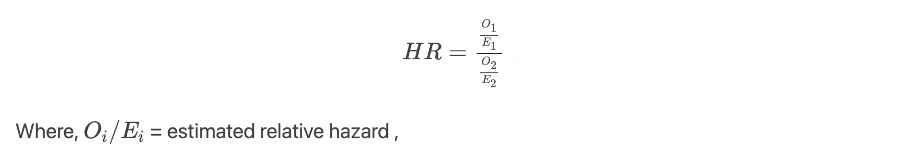HR is analogous to the odds ratio as in multiple logistic regression analysis. HR is useful in interpreting the results from the Cox proportional hazards (CPH) model.

#### Assumptions of Cox proportional hazards (CPH) model

• Hazard ratio (HR) is constant over time
• There should be a linear relationship between the log of hazard ratio and independent variables
• The independent variables should be independent of survival times i.e. independent variables should not change with time

#### Cox proportional hazards (CPH) model

Cox proportional hazards (CPH) model for X independent variables is given as,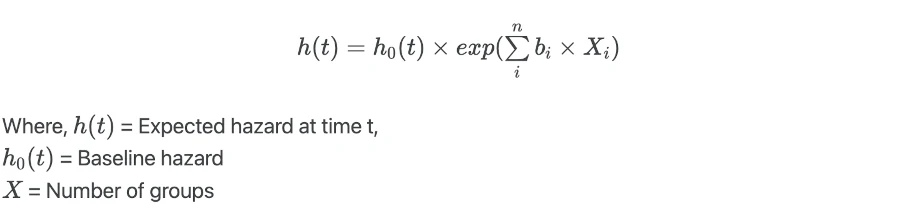When there is no effect of independent variables (X = 0),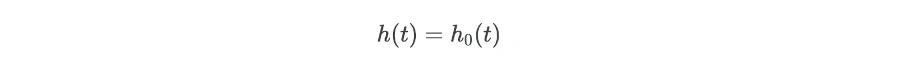The regression coefficients (bi) are important parameters for explaining the summary of the results. To get regression coefficients (bi) from regression model take log of hazard ratios,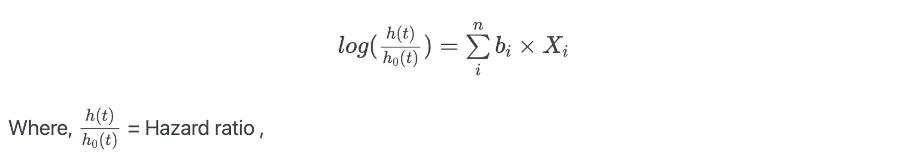From this function, the quantity exp(bi) is estimated. exp(bi) is the antilog of the estimated regression coefficient (bi). exp(bi) is also known as the hazard ratio. The regression coefficient (bi) describes the amount of change in the hazard ratio for one unit change in Xi whilst all other independent variables are constant.

## Perform survival analysis in R

We will use the survival, survminer and tidyverse packages to analyze data for survival analysis.

### Dataset

To perform survival analysis, we will use the hypothetical dataset. This dataset contains 15 patients with their survival times, outcome (1=death, 0=censored), treatments (drug_1 and drug_2), and age of the patients. The censored patients can be survived or dropped of from the study.

library(tidyverse)
# output
patient survival_time_days outcome treatment age_years
<dbl>              <dbl>   <dbl> <chr>         <dbl>
1       1                  1       1 drug_2           75
2       2                  1       1 drug_2           79


### Kaplan–Meier survival analysis

For Kaplan–Meier analysis, you need three key variables i.e. survival time, status at survival time (event of interest), and treatment groups of patients.

To perform Kaplan–Meier analysis in R, we will use the survfit() function from the survival package.

First, create a survival object with survival time and outcome. In a survival object, the event parameter must be logical (T/F) where T=death, or numeric (0/1) where 1=death.

library("survival")

surv = Surv(time = df$survival_time_days, event = df$outcome)
surv
# output
  1   1   4   5   6+  8   9+  9  12  15+ 22  25+ 37  55  72+


In above output, the + sign indicates that survival time was censored

Now, we will compute the survival probability for both drug treatments using survfit() function. In survfit() function, we use formula parameter which includes Surv object as response (left side) and treatments (right side) for estimating survival probability. If there is single treatment (single survival curve), the right-hand side should ~ 1.

fit <- survfit(formula = surv ~ treatment, data = df)
fit
# output
Call: survfit(formula = surv ~ treatment, data = df)

n events median 0.95LCL 0.95UCL
treatment=drug_1 7      4     37      12      NA
treatment=drug_2 8      6      7       4      NA


Get a summary of survival probability for each survival time,

summary(fit)
# output
Call: survfit(formula = surv ~ treatment, data = df)

treatment=drug_1
time n.risk n.event survival std.err lower 95% CI upper 95% CI
8      7       1    0.857   0.132       0.6334            1
12      6       1    0.714   0.171       0.4471            1
37      3       1    0.476   0.225       0.1884            1
55      2       1    0.238   0.203       0.0449            1

treatment=drug_2
time n.risk n.event survival std.err lower 95% CI upper 95% CI
1      8       2    0.750   0.153        0.503        1.000
4      6       1    0.625   0.171        0.365        1.000
5      5       1    0.500   0.177        0.250        1.000
9      3       1    0.333   0.180        0.116        0.961
22      1       1    0.000     NaN           NA           NA


Visualize the Kaplan–Meier survival curve for both treatments (drug_1 and drug_2). We will use the ggsurvplot() function from survminer package.

library("survminer")
ggsurvplot(fit = fit, pval = TRUE, surv.median.line = "hv",
xlab = "Survival time (Days)", ylab = "Survival probability")

# with confidence interval
ggsurvplot(fit = fit, pval = TRUE, surv.median.line = "hv", conf.int =TRUE,
xlab = "Survival time (Days)", ylab = "Survival probability")


Generated survival plot,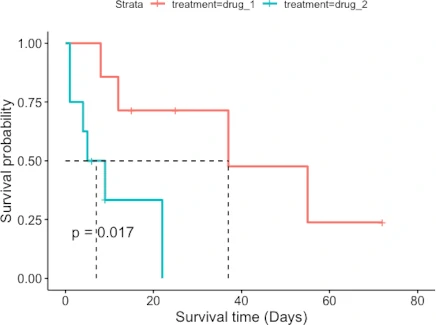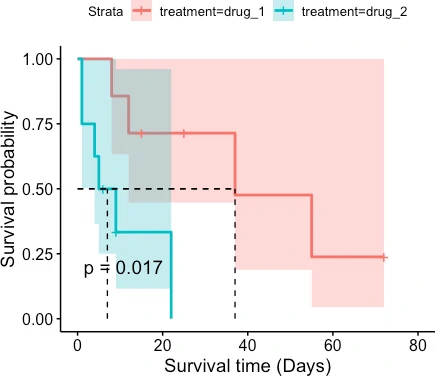The patient survival rate is higher for drug_1 treatment than drug_2 treatment. Similarly, the median survival time (time at which survival probability is 50%) is higher for patients taking drug_1 treatment (37 days) than drug_2 treatment (7 days).

### Log rank test to compare survival curves

Log rank test is a nonparametric test, which is used for checking whether there are differences between two or more survival curves. Log rank test can be performed using the survdiff() available in the survival package.

log_rank_test <- survdiff(formula = surv ~ treatment, data = df)
log_rank_test
# output
Call:
survdiff(formula = surv ~ treatment, data = df)

N Observed Expected (O-E)^2/E (O-E)^2/V
treatment=drug_1 7        4     7.08      1.34      5.68
treatment=drug_2 8        6     2.92      3.25      5.68

Chisq= 5.7  on 1 degrees of freedom, p= 0.02


The p value obtained from Log rank test is significant [χ2 = 5.7, p = 0.02] and indicates that there are significant differences between the survival curves for drug_1 and drug_2 treatments.

### Cox’s proportional hazards (CPH) model

In Kaplan–Meier, we use only one variable (event of interest) for estimating the survival curves. Log rank test compares two or more survival curves and does not consider additional independent variables.

Cox’s proportional hazards model (Cox regression) considers additional independent variables (covariates) for estimating the differences between the survival curves. Cox regression model is similar to multiple regression model where multiple independent variables are considered in the analysis.

Run Cox’s proportional hazards model with treatment and age as independent variables,

cox_reg_model <- coxph(formula = surv ~ treatment + age_years, data = df)
summary(cox_reg_model)
# output
Call:
coxph(formula = surv ~ treatment + age_years, data = df)

n= 15, number of events= 10

coef exp(coef) se(coef)     z Pr(>|z|)
treatmentdrug_2 1.88484   6.58531  0.96833 1.946  0.05160 .
age_years       0.21739   1.24283  0.08429 2.579  0.00991 **
---
Signif. codes:  0 ‘***’ 0.001 ‘**’ 0.01 ‘*’ 0.05 ‘.’ 0.1 ‘ ’ 1

exp(coef) exp(-coef) lower .95 upper .95
treatmentdrug_2     6.585     0.1519     0.987    43.936
age_years           1.243     0.8046     1.054     1.466

Concordance= 0.873  (se = 0.034 )
Likelihood ratio test= 14.41  on 2 df,   p=7e-04
Wald test            = 9.03  on 2 df,   p=0.01
Score (logrank) test = 12.61  on 2 df,   p=0.002


The results from Cox’s proportional hazards (CPH) model indicate that the effect of treatment (considering age) on patient survival was not significant (p > 0.05). However, the age of the patient has a significant effect (p < 0.05) on patient survival time.

The exp(coef) and exp(-coef) refers to the hazard ratio and inverse of the hazard ratio, respectively. The hazard ratio for treatment is 6.585 (reference group is drug_1) which indicates that patients receiving drug_2 treatment are 6.58 likely to experience the event of interest (death) than patients receiving drug_1.

The hazard ratio for age is 1.243. This indicates that if the patient increases by one year, the risk of an event of interest (death) increases by a factor of 1.243.

## Enhance your skills with courses on survival analysis

If you have any questions, comments or recommendations, please email me at reneshbe@gmail.com